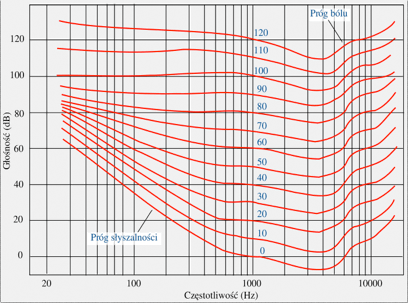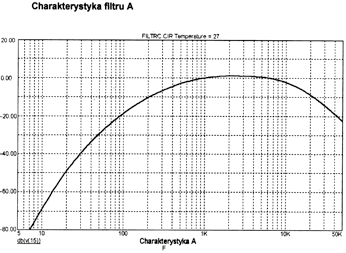# Basics of acoustics

Noise

Noise is defined as all unwanted, unpleasant and annoying sounds that affect humans. Being harmful to life and health, noise is recognized as an important determinant of environmental quality.

Sound

TThe acoustic wave propagating in the elastic medium or the auditory sensation caused by this wave. It is assumed that the human can detect sounds with frequencies ranging from 16 Hz to 20 kHz. Low-frequency vibrations are referred to as infrasound while the ones with higher frequency as ultrasound. The most easily heard sounds are the ones ranging from 3 to 4 kHz. The physical aspects of sound are its spectrum, level, duration of sound and changes in time.

The characteristics in the figure below show the sensitivity curves of the human ear depending on the frequency and volume level.Level L (dB)
The term denotes 10 decimal logarithms of the ratio of the square of the sound pressure to the square of the reference sound pressure of 2 * 10-5 Pa. The reference level roughly corresponds to the most silent sound detectable by the human ear. The higher the sound level expressed in dB is, the louder the sound itself is. It can be assumed that the change by 2 dB is barely noticeable, the change by 6 dB is clearly audible, which means sound twice as loud.

Frequency f (Hz):
It refers to periodical or cyclical phenomena like a sound wave. The frequency of this phenomenon determines how many times it is repeated within one second. The unit of frequency is Hertz (Hz) corresponding to 1 cycle per second.

A frequency weighting
A correction curve is an inverted isophonic curve for 40 phons that is used in acoustic measurements in order to take into account the properties of the human ear which can hear best in the medium frequency range while worse in low and high ranges. The correction curve shape is shown below.Conventional meter: a meter in which the measured quantity is the sound level determined by the exponential averaging method;

Integral-averaging meter: a meter in which the equivalent sound level is measured;

Integration meter: a meter in which the sound exposure level is measured;

Explanation of some quantities measured in acoustics in a descriptive way.

LAS current RMS sound level, A-weighted and S time-weighted.
LASmx maximum RMS sound level, A-weighted and S time-weighted.
LAF current RMS sound level, A-weighted and F time-weighted.
LAFmn minimum RMS sound level, A-weighted and F time-weighted.
LCPk maximum peak value of sound level, C-weighted.
LAeq equivalent A-weighted sound level. This is a substitute constant sound level that produces the same energy effect as the one produced by any variable sound at the same time.
LAeq1s equivalent A-weighted sound level, measured in 1 second.
LAE sound exposure level. This is a substitute constant sound level that would produce within 1s an energetic effect equal to the one produced within the measured time limit.
LAEX,8h sound exposure level related to an 8-hour working day.

Mathematical representation of acoustic quantities

Sound pressure level L: value expressed in decibels, determined according to the formula:

L = 10 log (p / p0)2

where:
p - measured effective value of sound pressure expressed in pascals,
p0 - sound pressure reference value equal to 2 × 10-5Pa;

Maximum C-weighted peak sound level during observation time LCMPk : value expressed in decibels, determined by the formula:

LCMPk = 10 log [max |pC(t)| /p0]2

where:
pC(t) - instantaneous C-weighted sound pressure, expressed in pascals;

Equivalent A sound level LAeq: value expressed in decibels, determined by the formula:

L = 10 log {1 / T ∫0T [pA (t) / p0] 2 dt}

where:
T - measurement time expressed in seconds;
pA (t) - instantaneous A-weighted sound pressure, expressed in pascals;

Sound exposure EA: value expressed in pascals squared times second, determined according to the formula:

EA = ∫0T [pA (t)]2 dt

Sound exposure level LAE: value expressed in decibels, determined by the formula:

LAE = 10 log (EA / p02 T0)

where:
T0 - reference time equal to 1s;